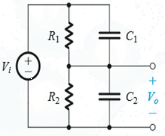Courses

# Test: Frequency Response of Amplifier

## 10 Questions MCQ Test Analog Circuits | Test: Frequency Response of Amplifier

Description
This mock test of Test: Frequency Response of Amplifier for Electronics and Communication Engineering (ECE) helps you for every Electronics and Communication Engineering (ECE) entrance exam. This contains 10 Multiple Choice Questions for Electronics and Communication Engineering (ECE) Test: Frequency Response of Amplifier (mcq) to study with solutions a complete question bank. The solved questions answers in this Test: Frequency Response of Amplifier quiz give you a good mix of easy questions and tough questions. Electronics and Communication Engineering (ECE) students definitely take this Test: Frequency Response of Amplifier exercise for a better result in the exam. You can find other Test: Frequency Response of Amplifier extra questions, long questions & short questions for Electronics and Communication Engineering (ECE) on EduRev as well by searching above.
QUESTION: 1

### Consider a voltage amplifier having a frequency response of the low-pass STC type with a dc gain of 60 dB and a 3-dB frequency of 1000 Hz. Then the gain db at

Solution:

Use standard formulas for frequency response and voltage gain.

QUESTION: 2

### STC networks can be classified into two categories: low-pass (LP) and high-pass (HP). Then which of the following is true?

Solution:

By definition a LP network allows dc current (or low frequency current) and an LP network does the opposite, that is, allows high frequency ac current.

QUESTION: 3

### Single-time-constant (STC) networks are those networks that are composed of, or can be reduced to

Solution:

STC has only one reactive component and one resistive component.

QUESTION: 4

The signal whose waveform is not effected by a linear circuit is

Solution:

Only sine/cosine wave are not affected by a linear circuit while all other waveforms are affected by a linear circuit.

QUESTION: 5

Which of the following is not a classification of amplifiers on the basis of their frequency response?

Solution:

None of the options provided are correct.

QUESTION: 6

General representation of the frequency response curve is called

Solution:

General representation of frequency response curves are called Bode plot. Bode plots are also called semi logarithmic plots since they have logarithmic values values on one of the axes.

QUESTION: 7

Under what condition can the circuit shown be called a compensated attenuator.​Solution:
QUESTION: 8

When a circuit is called compensated attenuator?

Solution:

Transfer function does not has frequency in its mathematical formula.

QUESTION: 9

Which of the following is true?

Solution:

Both the statements are false.

QUESTION: 10

Which of the following is true?

Solution:

These all are practical applications of different types of amplifiers.